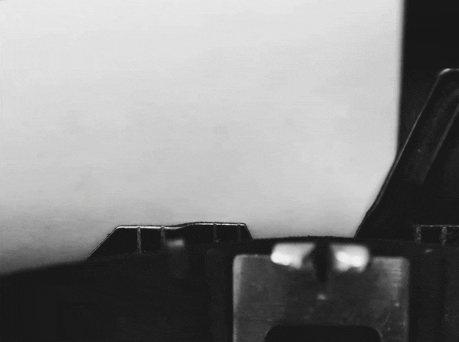## What is the level 5 Google Foobar challenge?

There is only one challenge at this level. We have 22 days with it. Too much for a lazy person like me.

#### challenge I - Expanding Nebula:

You’ve escaped Commander Lambda’s exploding space station along with numerous escape pods full of bunnies. But – oh no! – one of the escape pods has flown into a nearby nebula, causing you to lose track of it. You start monitoring the nebula, but unfortunately, just a moment too late to find where the pod went. However, you do find that the gas of the steadily expanding nebula follows a simple pattern, meaning that you should be able to determine the previous state of the gas and narrow down where you might find the pod.

From the scans of the nebula, you have found that it is very flat and distributed in distinct patches, so you can model it as a 2D grid. You find that the current existence of gas in a cell of the grid is determined exactly by its 4 nearby cells, specifically, (1) that cell, (2) the cell below it, (3) the cell to the right of it, and (4) the cell below and to the right of it. If, in the current state, exactly 1 of those 4 cells in the 2x2 block has gas, then it will also have gas in the next state. Otherwise, the cell will be empty in the next state.

For example, let’s say the previous state of the grid (p) was:

 ``````1 2 3 4 `````` ``````.O.. ..O. ...O O... ``````

To see how this grid will change to become the current grid (c) over the next time step, consider the 2x2 blocks of cells around each cell. Of the 2x2 block of [p, p, p, p], only p has gas in it, which means this 2x2 block would become cell c with gas in the next time step:

 ``````1 2 `````` ``````.O -> O .. ``````

Likewise, in the next 2x2 block to the right consisting of [p, p, p, p], two of the containing cells have gas, so in the next state of the grid, c will NOT have gas:

 ``````1 2 `````` ``````O. -> . .O ``````

Following this pattern to its conclusion, from the previous state p, the current state of the grid c will be:

 ``````1 2 3 `````` ``````O.O .O. O.O ``````

Note that the resulting output will have 1 fewer row and column, since the bottom and rightmost cells do not have a cell below and to the right of them, respectively. Write a function solution(g) where g is an array of array of bools saying whether there is gas in each cell (the current scan of the nebula), and return an int with the number of possible previous states that could have resulted in that grid after 1 time step. For instance, if the function were given the current state c above, it would deduce that the possible previous states were p (given above) as well as its horizontal and vertical reflections, and would return 4. The width of the grid will be between 3 and 50 inclusive, and the height of the grid will be between 3 and 9 inclusive. The solution will always be less than one billion (10^9).

 ``````1 2 3 4 5 `````` ``````Solution.solution({{true, false, true}, {false, true, false}, {true, false, true}}) 4 Solution.solution({{true, false, true, false, false, true, true, true}, {true, false, true, false, false, false, true, false}, {true, true, true, false, false, false, true, false}, {true, false, true, false, false, false, true, false}, {true, false, true, false, false, true, true, true}} 254 ``````

## How did I solve the level 5 Google Foobar challenge?

#### challenge I - Expanding Nebula:

Have you heard of Game of Life? To solve this level you need to know about Cellular Automata

Of course, bro, don’t expect anything from me. I gave up trying to understand and solve the challenge since previous levels

The solution was revealed from this source

### solution.py:

#### challenge I - Expanding Nebula:

 `````` 1 2 3 4 5 6 7 8 9 10 11 12 13 14 15 16 17 18 19 20 21 22 23 24 25 26 27 28 29 30 31 32 33 34 35 36 37 38 `````` ``````from collections import defaultdict def generate(c1,c2,bitlen): a = c1 & ~(1<> 1 d = c2 >> 1 return (a&~b&~c&~d) | (~a&b&~c&~d) | (~a&~b&c&~d) | (~a&~b&~c&d) def build_map(n, nums): mapping = defaultdict(set) nums = set(nums) for i in range(1<<(n+1)): for j in range(1<<(n+1)): generation = generate(i,j,n) if generation in nums: mapping[(generation, i)].add(j) return mapping def solution(g): g = list(zip(*g)) # transpose nrows = len(g) ncols = len(g) # turn map into numbers nums = [sum([1<

## The End

This is the screen when I submit the solution for the level 5 challenge``````1 2 3 4 5 6 7 8 9 `````` ``````import ast import base64 from itertools import cycle message = "FUAcHQwCCxRdXkVWTEkAHQ0OFUlLDl4GAwACAg4PGgRJRxRZQgkfGgIKBQoFSUsOXgAKCgEVGxtI QVRHCRALDx4LAwYKAwRJSw5eBA8EBwIZDQIEABMJWV9MSxsJAwcMCgsDCVVFSx4PBQ0BGxJJRxRZ Qh8NCAJIRE9GCAhBXkVWTEkQBgZORhM=" key = bytes("ngohoang.yell", "utf-8") decrypted_data = bytes(a ^ b for a, b in zip(base64.b64decode(message), cycle(key))) result = ast.literal_eval(decrypted_data.decode()) print(result) ``````

Result

 ``````1 2 3 4 5 6 7 8 `````` ``````{ 'success': 'great', 'colleague': 'esteemed', 'efforts': 'incredible', 'achievement': 'unlocked', 'rabbits': 'safe', 'foo': 'win!' } ``````

That’s all! You are all free now, let’s spend time doing other things!

Congratulations 👏🎉Are you happy when it’s over? If not, what are you expecting? You want more challenges, right?

Try typing `request` and see the surprise 😈